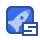• ### Il2CppDumper 6.4.12 源码,程序

Il2CppDumper 最新版本v6.4.12, 包含源码, 可执行程序, C#源码 Unity il2cpp逆向工程 功能 还原DLL文件（不包含代码），可用于提取MonoBehaviour和MonoScript 支持ELF, ELF64, Mach-O, PE, NSO和WASM格式 支持Unity 5.3 - 2020 生成IDA和Ghidra的脚本，帮助IDA和Ghidra更好的分析il2cpp文件 生成结构体头文件 支持从内存dump的libil2cpp.so文件以绕过保护 支持绕过简单的PE保护

2020-08-30
50
• ### CurveFitter.java

2018-07-19 最新更新, 主要是曲线拟合算法源码, 包含大多数常用的方程算法, 包括如下: "y = a+bx", "y = a+bx+cx^2", "y = a+bx+cx^2+dx^3", "y = a+bx+cx^2+dx^3+ex^4", "y = a*exp(bx)", "y = a*x^b", "y = a*ln(bx)", "y = d+(a-d)/(1+(x/c)^b)", "y = b*(x-a)^c*exp(-(x-a)/d)", "y = a+b*ln(x-c)", "x = d+(a-d)/(1+(y/c)^b) [y = c*((x-a)/(d-x))^(1/b)]", "y = a*exp(-bx) + c", "y = a + (b-a)*exp(-(x-c)*(x-c)/(2*d*d))", "y = a*(1-exp(-b*x)) + c", "y = c*((x-a)/(d-x))^(1/b)", "y = a*exp(bx)", "y = a*x^b", "y = a+bx+cx^2+dx^3+ex^4+fx^5", "y = a+bx+cx^2+dx^3+ex^4+fx^5+gx^6", "y = a+bx+cx^2+dx^3+ex^4+fx^5+gx^6+hx^7", "y = a+bx+cx^2+dx^3+ex^4+fx^5+gx^6+hx^7+ix^8", "y = a*exp(-(x-b)*(x-b)/(2*c*c)))", "y = a*(1-exp(-b*x))", "y = a*(1-exp(-b*x))^c", "y = a+b*erf((x-c)/d)"

2020-08-15
50
• ### alglib-3.10.0.rar

ALGLIB is a cross-platform numerical analysis and data mining library. It supports several programming languages (C++, C#, Pascal, VBA) and several operating systems (Windows, *nix family). ALGLIB features include: Data analysis (classification/regression, including neural networks) Optimization and nonlinear solvers Interpolation and linear/nonlinear least-squares fitting Linear algebra (direct algorithms, EVD/SVD), direct and iterative linear solvers, Fast Fourier Transform and many other algorithms (numerical integration, ODEs, statistics, special functions)

2020-08-15
50
• ### Chemiluminescence_instrumentV3.rar

某个化学发光仪上位机完整源码, QT源码, 包括行业内一般的算法 如 四参数拟合算法( four Parameter Logistic )等其他拟合插值算法

2020-08-15
14
• ### PDB符号文件查看工具 symview,pdbxtract

PDB符号文件查看工具, 包含2个工具symview 和pdbxtract symview可查看文件所有原始信息 pdbxtract 可方便查看结构体等信息, 可导出头文件或xml

2020-06-06
50
• ### Intel B75芯片 PDF

BD82B75 是Intel 生产的南桥芯片, 所有的B75主板都是用这个芯片 属于Intel的 7系列 PCH Intel® 7 Series / C216 Chipset Family Platform Controller Hub (PCH) 如开发PCI硬件, 或监控硬件信息可用到该资料

2020-06-01
47
• ### NCT6779D 主板IO芯片pdf

NCT6779D 是Nuvoton生产的一款IO芯片 广泛用于电脑主板, 如ASUS B75主板 利用该资料可控制或获取主板许多信息, 如监控信息:温度, 电压, 风扇等 另外还有其他等

2020-06-01
44
• ### FontCreator13.rar

FontCreator 是一款专业的字体编辑器，它允许您创建和编辑TrueType和OpenType字体，软件拥有排版和平面设计师所需要的强大绘图工具，以及一个直观的界面，让初学者可以立即变得富有成效。

2020-04-12
49
• ### CodeYh.ttf

自己制作的由consola和雅黑字体组合成的字体, 用于代码字体 网上其他各种字体有各种问题, 制作笔记: 写代码使用的等宽字体中文显示是宋体, 很不好看, 可以用多个方法 A. 使用注册表的SystemLink 计算机\HKEY_LOCAL_MACHINE\SOFTWARE\Microsoft\Windows NT\CurrentVersion\FontLink\SystemLink Consolas="MSYH.TTF,118,80\\r\\n" 118,80 控制比例,高度 使用该方法部分字符宽度出现问题, 如全角的空格(\$3000)等 B. 使用FontCreator修改字体 1. 微软雅黑字体内部使用高清轮廓, 对字符任何修改导致丢失高清数据, 导致显示有些偏差, 很不美观, 只能修改英文字符, 中文要保留不能动 2. 行距可以在字体的度量修改上下,以及间距 3. 等宽字体需要修改特性的字族类为2 比例为9 4. 字符复制需要先排序再复制, 否则复制的顺序不对 5. 字体的宽度是由字符度量的左右 6. 部分版本生成的字体tab字符的宽度控制不对, 造成错位 7. 安装的字体如果放在特殊位置如虚拟磁盘, 控制面板将看不到该字体 8. 全角的空格问题一样有, FontCreator 必须要V13以上才可以避免这个问题, 而且字体安装后需要注销或重启才能看到这个效果, 或者把字体另外改名 9. 部分文字组合会导致字距变换, 需要在菜单Opentrue设计者删除对应的字距调整kern

2020-04-12
10
• ### ollydumpX插件, 显示xmm寄存器等

功能: 1.显示代码对应的xmm寄存器数据 2.OllyDump使用时出现 NtdllDefWindowProc_A 导入表错误 3.X64系统启动exe时出现异常EXCEPTION_SINGLE_STEP 4.自动对mswsock.dll文件的WSP系列函数命名添加标签 5.CPU信息,监视,日志窗体添加代码支持快捷复制Ctrl+C, Ctrl+A 6.CPU_DUMP添加菜单可以复制字符串

2020-03-08
50
• 72
资源
• 263
粉丝
•等级